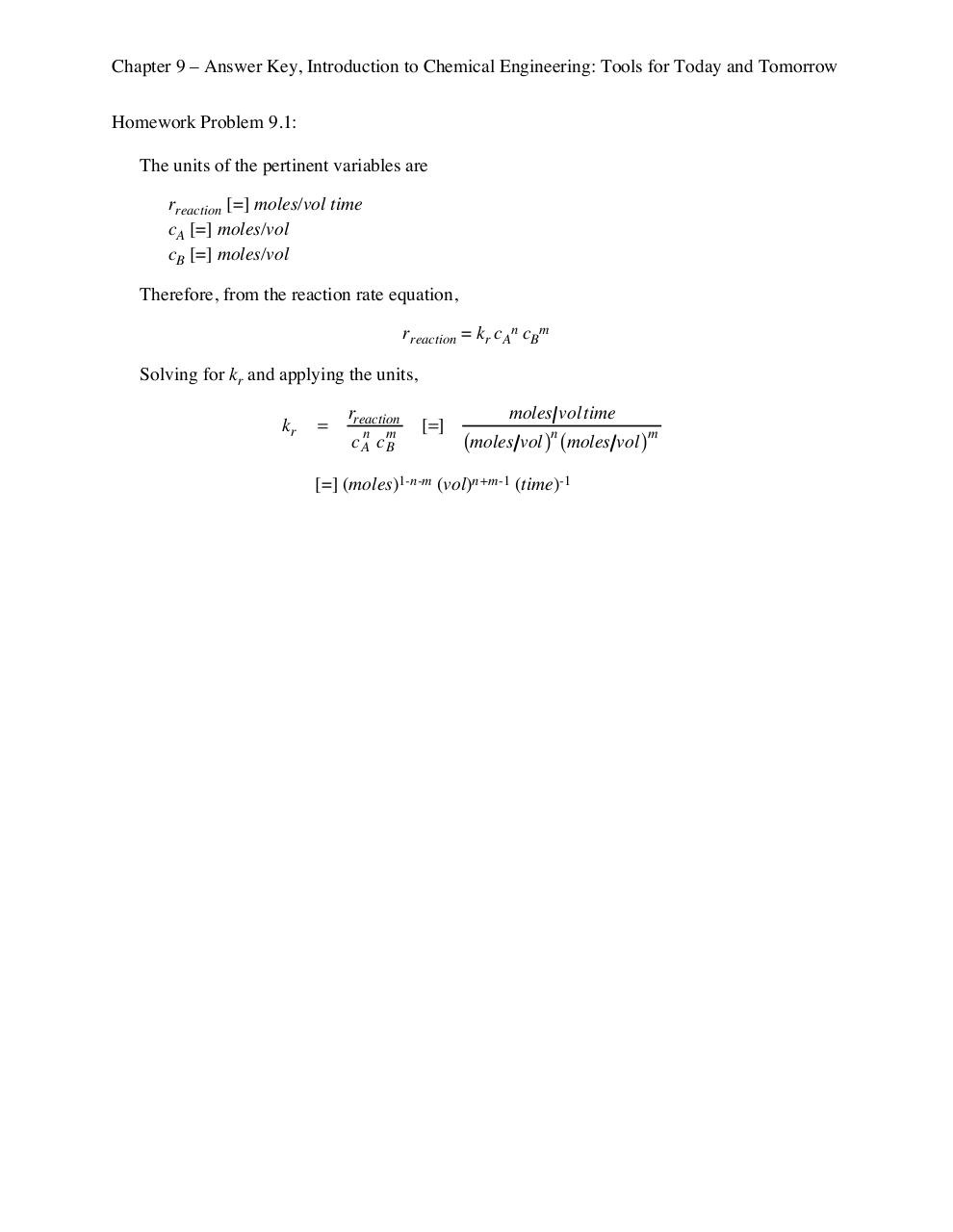# introduction to chemical engineering ch (9).pdfPage 1 2 3 45614

#### Text preview

Chapter 9 – Answer Key, Introduction to Chemical Engineering: Tools for Today and Tomorrow
Homework Problem 9.1:
The units of the pertinent variables are
rreaction [=] moles/vol time
cA [=] moles/vol
cB [=] moles/vol
Therefore, from the reaction rate equation,
rreaction = kr cAn cBm
Solving for kr and applying the units,

kr

=

rreaction
c An c m
B

[=]

moles vol time
(moles vol )n (moles vol )m

[=] (moles)1-n-m (vol)n+m-1 (time)-1